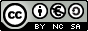Secondary Education INDEX - The multiples of a number. - The factors of a number. - Take the factors. - Find the factors of a number. - A game with multiples and factors. - The Sieve of Erathostenes. - Composite or prime numbers. - Prime factor decomposition of a number. - The highest common factor of two numbers. - The least common multiple of two numbers. - The least common multiple of three numbers.
Multiples and factors. Prime numbers
INTRODUCTION
These activities are designed as games and as small mathematical investigations; before doing them, students must have already studied all the concepts and principles mentioned in them.

These activities raise questions aimed to be starting points for small investigations done by students. The teacher may suggest any other activity and can also make the most of the ideas hinted at by the students themselves.

OBJECTIVES
• To reinforce the principles previously studied in class:
-
The multiple of a number.
- The factor of a number.
- Prime number.
- Composite number.
- The factor decomposition of a number.
- The highest common factor of several numbers.
-
The least common multiple of several numbers.
• To learn new calculation strategies.
• To appreciate the recreational and competitive aspects of Maths.
• To foster individual math investigation by the student as a means of learning.
 Eduardo Barbero CorralSpanish Ministry of Education. Year 2007